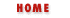XML, the Perl Way7. Advanced featuresTable of Content9. Reference

## 8. Under the hood

Now let's have a look under the hood at some of the things that go on in XML::Twig from a developer stand point.

### 8.1 Speedup

I think one of the most interesting feature of XML::Twig is the optimization step that takes place when the module is installed.

The module is written in pure OO style, whith accessors for every fields of objects, even inside the module. But as we all know method calls are expensive. So an optimization pass replaces method calls by hash accesses if possible.

For example \$elt->parent is replaced by \$elt->{parent} and \$elt->set_parent( \$parent) is replaced by \$elt->{parent}= \$parent.

The speedup is pretty simple, just a bunch of substitutions, and certainly not foolproof (it would crash miserably if I were to use brackets in the argument list of a method). It works pretty well though, and if it fails then the non-regression tests will catch the problem. It could be improved by using 5.6 new regexp to fix this.

 ```#!/bin/perl -p BEGIN { \$FIELD="parent|first_child|last_child|prev_sibling|next_sibling|pcdata|cdata|flushed"; } s/(\\$[a-z_]+)->del_(twig_current|flushed)/delete \$1\->{'\$2'}/g; s/(\\$[a-z_]+)->set_(twig_current|flushed)/\$1\->{'\$2'}=1/g; s/(\\$[a-z_]+)->set_(\$FIELD)\(([^)]*)\)/\$1\->\{'\$2'\}= \$3/g; s/(\\$[a-z_]+)->(\$FIELD)/\$1\->\{'\$2'\}/g; s/(\\$[a-z_]+)->set_atts\(([^)]*)\)/\$1\->\{'att'\}= \$2/g; s/(\\$[a-z_]+)->(atts)\(([^)]*)\)/\$1\->\{'att'\}/g; s/(\\$[a-z_]+)->append_(pcdata|cdata)\(([^)]*)\)/\$1\->\{\$2\}.= \$3/g; s/(\\$[a-z_]+)->gi/\\$XML::Twig::index2gi\[\$1\->{'gi'}\]/g; s/(\\$[a-z_]+)->id/\$1\->{'att'}->{\\$ID}/g; s/(\\$[a-z_]+)->att\(\s*([^)]+)\)/\$1\->{'att'}->\{\$2\}/g; s/(\\$[a-z_]+)->is_pcdata/(exists \$1\->{'pcdata'})/g; s/(\\$[a-z_]+)->is_cdata/(exists \$1\->{'cdata'})/g; ```

The result is an improvement of about 30% of the speed of the module.

Speedup could also be used to... speedup a production script, with the caveat that as XML::Twig implementation changes it might be necessary to re-run the tool with new versions of the module.

### 8.2 Element names "compression"

A minor optimization in XML::Twig is that element names, which are stored as hash values are replaced by an index in an array holding all names.

### 8.3 Failed optimizations

Not all attempts at optimizing XML::Twig succeded, so I think it might be useful for me to share at least my biggest failure in this area...

Twig elements are stored in hashes, one element per hash. In order to reduce the potential overhead of all too much memory being allocated for each one of them I tried to store elements in global arrays, each array storing one field for all the elements: instead of the parent of an element being stored in \$elt->{parent} it was stored in \$parent[\$elt], \$elt being a blessed scalar.

It did not work.

The twig was just as big and slower to access than the original version.

Oh well... there goes 2 days of work...7. Advanced featuresTable of Content9. Reference